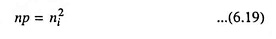## Mass Action Law in Semiconductor:

As already mentioned, concentration of free electrons and holes is always equal in an intrinsic semiconductor. When N-type impurity is added to an intrinsic semiconductor, the concentration of free electrons is increased (or the concentration of holes is reduced below the intrinsic value). Similarly addition of P-type impurity results in reduction of concentration of free electrons below the intrinsic value. Theoretical analysis reveals that under thermal equilibrium the product of concentration of free electrons and concentration of holes is constant and is independent of the amount of doping by donor and acceptor impurities. This is known as Mass Action Law in Semiconductor. Thus,where

• ni is the intrinsic concentration and is a function of temperature.

For an intrinsic semiconductor n = p = ni.

The above discussion reveals that the doping of impurity of either type in an intrinsic semiconductor causes increase in the conductivity and results in either free electrons or holes to dominate the situation.

This law is very important from the point of view that it enables us to determine minority carriers concentration. According to this law the addition of impurities to an intrinsic semiconductor increases the concentration of one type of carriers, which consequently become majority carriers and simultaneously decreases the concentration of the other carriers, which as a result become minority carriers.

Scroll to Top